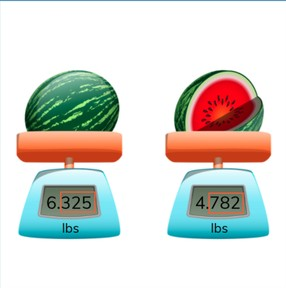Determine the difference between decimal numbers with 3 decimal places

# Determine the difference between decimal numbers with 3 decimal places

No account needed.8,000 schools use Gynzy92,000 teachers use Gynzy1,600,000 students use Gynzy

## General

Students learn to determine the difference between decimal numbers with three decimal places.

6.NS.B.3

## Relevance

It is useful to be able to determine difference, so you can tell which number is greater or less than the other. You can use this to determine which melon weighs more.

## Introduction

The interactive whiteboard shows a few addition problems with decimal numbers with 3 decimal places. Each subtraction problem is missing its second number. Students must determine which of the given numbers belong to each subtraction problem. They can be dragged to where they belong on the board.

## Development

Explain to students that if you want to determine the difference between two numbers, that you are looking to see how many more, or how many less one number is than the other. Show students that you can use a number line to determine the difference between two numbers, by counting up from the smaller number to the larger number. Show this in three steps. First you jump from the decimal number to the next whole number. Then you jump to the next whole number. Finally you jump to the decimal number again. Next show students that you can also make a subtraction problem using the two decimal numbers. For example you can use the numbers 38.765 and 15.231 to make the subtraction problem of 38.765- 15.231 to determine the difference. Remind students that this method does not work if the numbers after the decimal point of the second number (subtrahend) are greater than those of the first number (minuend). Ask students to solve some problems and to explain their strategy. Next discuss how to solve a decimal story problem. Remind students to first determine what kind of math problem they will make, then to determine which numbers are important, to say or write the problem, and finally to solve the problem. Solve one story problem as a class and then as students to solve the next decimal story problem individually or in pairs.

To check that students understand determining the difference with decimal numbers with 3 decimal places, you can ask the following questions:
- Which methods can you use to determine difference?
- Which subtraction problem would you make to determine the difference between 78.632 and 62.354?
- What are the steps to solving a story problem?

## Guided practice

Students are given subtraction problems with decimal numbers with 3 decimal places. They are asked to either select the correct answer from the multiple choice options given, or to fill in the correct answer. Students are also given decimal story problems to solve. Remind students to say or use the steps for solving the story problem.

## Closing

Repeat the learning goal and why it is important. The interactive whiteboard shows two scales with candy and a weight shown in decimal numbers. There are two kids on the board who tell what the difference between the weights is. Ask students to solve and determine which of the students is correct. Ask students to explain their strategy.

## Teaching tips

Students who have difficulty determining difference can be supported by making use of a number line. Ask them to break down the numbers into smaller parts so that moving on the number line is easier.

### The online teaching platform for interactive whiteboards and displays in schools

• Save time building lessons

• Manage the classroom more efficiently

• Increase student engagement